# 子程序

## 子程序调用

 A B 1 func /create an ID 2 >id="" 3 >A1.run(id=id+char(65+rand(26))) 4 return id 5 =func(A1,rand(3)+3)

A1为主格的代码块，即第1~4行中，根据所给的字符长度，随机生成一个大写字母构成的ID。其中B2将网格变量id设为空字符串。B3根据子程序主格中获得的字符长度，执行循环，每次在id中随机添加一个大写字母。在每次执行前，需要先将使用的网格参数id初始化。在B4中，用return语句将网格变量id的值返回。在A5中，用func函数调用子程序，在=func(A1,rand(3)+3)中，A1表示所调用子程序的主格，rand(3)+3计算出一个3~5的随机整数作为参数，传递给子程序执行计算。这样A5中可以得到一个由3~5个随机字符构成的字符串，如：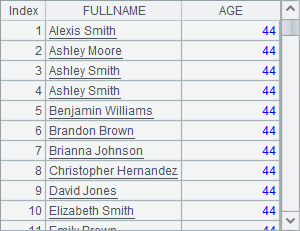A B 1 =5.(func(A2,2)) =10.(func(A2,rand(3)+3)) 2 func /create an ID 3 >id="" 4 >A2.run(id=id+char(65+rand(26))) 5 return id

A1中，随机生成5个由两个字母构成的字符串，作为产品名称的缩写。B1中，随机生成10个由3~5个字符构成的字符串，作为客户名称，A1B1中的结果如下：A B C 1 =create(Product,Customer) 0 2 func /create an ID 3 >id="" >A2.run(id=id+char(65+rand(26))) 4 return id 5 func 6 return [A7(A5),B7(B5)] 7 =5.(func(A2,2)) =10.(func(A2,rand(3)+3)) 8 for 100 =func(A5,rand(5)+1,rand(10)+1) >A1.insert(0,B8(1),B8(2))

A5为主格的子程序中，根据参数得到了1个产品名和1个客户名，在B6中将结果构成序列返回。另外B8调用子程序时，随机生成1个产品序号和1个客户序号，作为参数输入子程序。当调用子程序的参数多于1个时，会从主格起向右填充。

B8中，可以看到最后一次调用子程序时返回的结果：C8中使用结果时，再分别从序列中取出数据。第8行的程序随机生成100条数据插入A1中的序表，记录由产品名与客户名构成。执行后，A1中序表如下：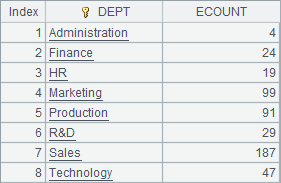A B C 1 =create(OID,Product, Customer,Amount) 0 2 func /create an ID 3 >id="" >A2.run(id=id+char(65+rand(26))) 4 return id 5 func /add a record 6 >B1=B1+1 =(rand(1000)+1)*100 7 >A1.insert(0,B1,A5,B5,C6) 8 =5.(func(A2,2)) =10.(func(A2,rand(3)+3)) 9 for 100 =A8(rand(5)+1) =B8(rand(10)+1) 10 >func(A5,B9,C9)

A2中的子程序和前面例子中的相同。A5中的子程序并没有return语句，此时子程序没有返回值，每次运行时只是在A1的序表中添加一条记录。因此，在B10调用A5中的子程序时，可以用>开头，子程序的调用会执行到A5的代码块结束为止。

A9中，循环生成100条测试数据，填入A1的序表中，结果如下：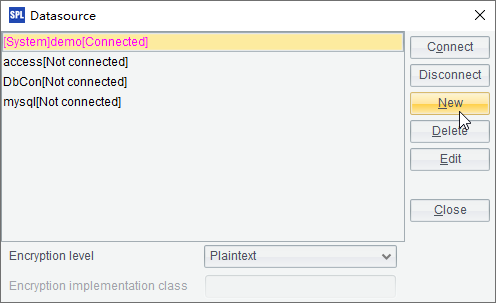A B C 1 func 2 if A1<=0 return 1 3 else >A5=A5+string(A1)+";" 4 return A1*func(A1,A1-1) 5 ="" 6 =func(A1,12)

A1的子程序中，根据传入的数据判断，当其大于0时，在C4中递归调用自身，计算阶乘。A6中计算12的阶乘如下：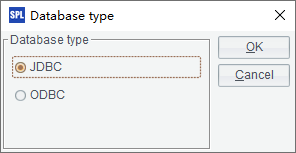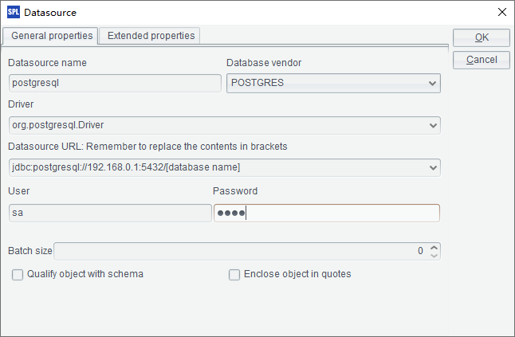A B C 1 func 2 =A1\B1 =A1%B1 3 if C2==0 return B1 4 else return func(A1,B1,C2) 5 =func(A1,4557,5115)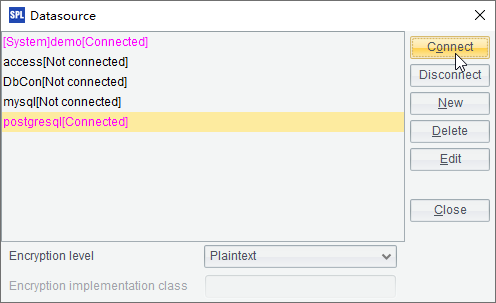A B C 1 func /create an ID 2 >id="" >A1.run(id=id+char(65+rand(26))) 3 =id Test 4 =5.(func(A1,2)) =10.(func(A1,rand(3)+3))

## 跨网格调用

 A B 1 /create an ID 2 >size.run(A2=A2+char(65+rand(26))) 3 return A2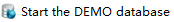A 1 =call("D:\\files\\createID.dfx",rand(3)+3) 2 =call("createID.dfx",rand(3)+3)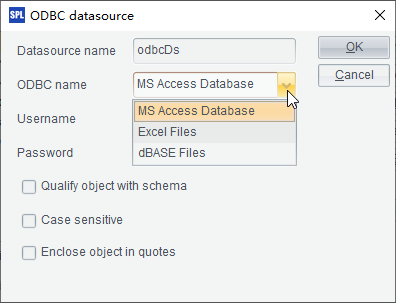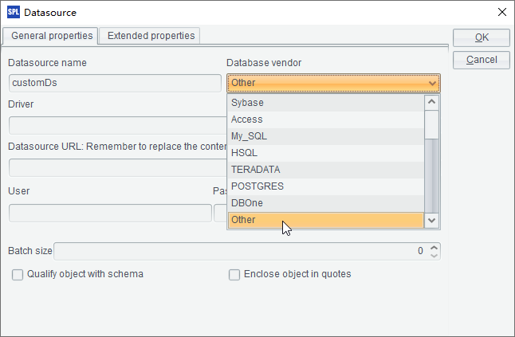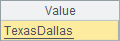<Esproc>

<dfxPathList>

<dfxPath>E:\tools\raqsoft\esProc\demo\Case\Structural</dfxPath>

<dfxPath>D:\files\txt</dfxPath>

</dfxPathList>

<mainPath>D:\files\demo</mainPath>

</Esproc>

 A B 1 /find a PNAME and a CNAME 2 =rand(PNames.len())+1 =rand(CNames.len())+1 3 return PNames(A2),CNames(B2)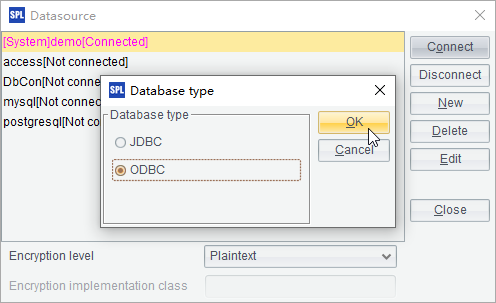A B 1 =create(Product,Customer) 2 =5.(call("createID.dfx ",2)) =10.(call("createID.dfx ",rand(3)+3)) 3 for 100 =call("findNames.dfx",A2,B2) 4 >A1.insert(0,B3(1),B3(2))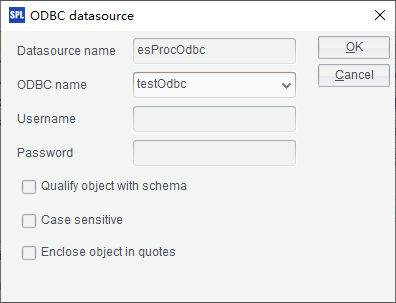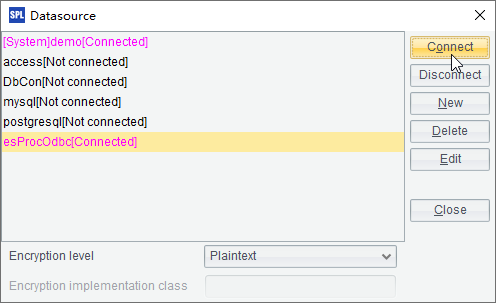A B 1 /add a record 2 =Table.len()+1 =(rand(1000)+1)*100 3 >Table.insert(0,A2,PNAME,CNAME,B2)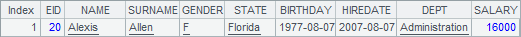A B 1 =create(OID,Product,Customer,Amount) 2 =5.(call("createID.dfx ",2)) =10.(call("createID.dfx ",rand(3)+3)) 3 for 100 =call("findNames.dfx",A2,B2) 4 >call("addRecord.dfx",B3(1),B3(2),A1)